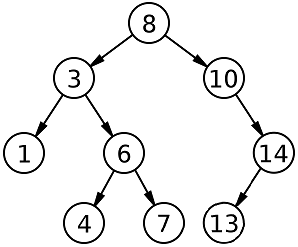Problem title
Difficulty
Avg time to solve

K Most Frequent Words
Moderate
36 mins
Best Time to Buy and Sell
Moderate
20 mins
Stack using queue
Moderate
25 mins
Deepest Leaves Sum
Easy
15 mins
Rotate matrix to the right
Moderate
20 mins
Subarray with equal occurrences
Moderate
15 mins
Max GCD Pair
Moderate
35 mins
Easy
15 mins
Container with Maximum Water
Easy
10 mins
Is SubSequence
Easy
10 mins8

# Predecessor and Successor In BST

Difficulty: MEDIUM
Avg. time to solve
25 min
Success Rate
65%

Problem Statement

#### Your task is to find the inorder successor and predecessor of the given X. Formally, print an array/list containing the inorder predecessor and successor in the same order.

##### For Example:
``````For the BST given below:
````````````The inorder predecessor of 6 is 4.
The inorder successor of 6 is 7.
The inorder predecessor of 10 is 8.
The inorder successor of 10 is 13.
``````

#### Note:

``````If there is no inorder predecessor or successor of 'X', then add -1 to the answer vector in its place.
``````
##### Input Format:
``````The first line of input contains a single integer 'T', representing the number of test cases or queries to be run.

Then the 'T' test cases follow:

The first line of each test case contains elements in the level order form.
The line consists of values of nodes separated by a single space. In case a node is null, we take -1 in its place. Refer example given below for more clarity.

The second line of each test case contains a single integer 'X'.
``````

#### Example:

``````The input for the tree depicted in the below image will be:
````````````1
2 3
4 -1 5 6
-1 7 -1 -1 -1 -1
-1 -1
``````

#### Explanation :

``````Level 1 :
The root node of the tree is 1

Level 2 :
Left child of 1 = 2
Right child of 1 = 3

Level 3 :
Left child of 2 = 4
Right child of 2 = null (-1)
Left child of 3 = 5
Right child of 3 = 6

Level 4 :
Left child of 4 = null (-1)
Right child of 4 = 7
Left child of 5 = null (-1)
Right child of 5 = null (-1)
Left child of 6 = null (-1)
Right child of 6 = null (-1)

Level 5 :
Left child of 7 = null (-1)
Right child of 7 = null (-1)

The first not-null node(of the previous level) is treated as the parent of the first two nodes of the current level. The second not-null node (of the previous level) is treated as the parent node for the next two nodes of the current level and so on.
The input ends when all nodes at the last level are null(-1).
``````
##### Note :
``````The above format was just to provide clarity on how the input is formed for a given tree.
The sequence will be put together in a single line separated by a single space. Hence, for the above-depicted tree, the input will be given as:

1 2 3 4 -1 5 6 -1 7 -1 -1 -1 -1 -1 -1
``````
##### Output Format:
``````Print a single line containing the inorder predecessor and successor of 'X' separated by a single space.

The output of each test case is printed in a separate line.
``````

#### Note:

``````You do not need to print anything, it has already been taken care of. Just implement the given function.
``````
##### Constraints:
``````1 <= T <= 5
1 <= N <= 5000
0 <= DATA <= 10^9
0 <= X <= 10^9

Where 'DATA' is the value of any node in the BST.

Time Limit: 1sec
``````
##### Sample Input 1:
``````1
8 5 10 2 6 -1 -1 -1 -1 -1 7 -1 -1
5
``````
##### Sample Output 1:
``````2 6
``````

#### Explanation of the Sample Input 1:

``````For the given ‘X’ = 5, according to the inorder view, 2 is the parent of the left subtree and 6 is the parent of the right subtree, hence 2 and 6 are inorder predecessors and successor respectively.
``````
##### Sample Input 2:
``````2
8 5 10 2 6 -1 -1 -1 -1 -1 7 -1 -1
6
8 5 10 2 6 -1 -1 -1 -1 -1 7 -1 -1
2
``````
##### Sample Output 2:
``````5 7
-1 5
``````Console